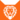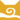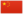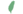# 一星

Onestar狮族 (Q646)风族 学徒风族 武士风族 副族长
（继任自泥掌 (Q2968)风族 族长
（继任自高星 (Q2621)小一 Onekit  小一
 一爪非官 Onepaw  一掌非官
 一根须、一须 Onewhisker  一鬚
 一星 Onestar  一星

Q3404：风族公猫，自《预言开始》系列登场的角色

## 细节

### 外貌

• 他是一只身形娇小纤细而:3矫健、:197琥珀色眼睛、:139毛色斑驳:64的淡:66棕色虎斑公猫，:猫物表右耳后有一道伤疤，[注 1]脚掌很小。:197
• 他生前有着泛灰的口鼻，:42但在加入星族后已变回年轻健壮的样子。:82

## 语录

—— 一根须对火心，《寒冰烈火》，第6章

—— 一根须对火心展现同情，《风起云涌》，第30章

—— 一星警告松鸦羽，《群星之战》，第75-76页

—— 松针爪和滑爪，关于一星，《学徒探索》，第45页

—— 一星的自白，《天空破碎》，第251页

—— 隼飞对一星，在他坦言自己害怕加入黑森林后，《天空破碎》，第258页

—— 兔星对族群，在森林大会上，《极夜无光》，第2章

## 登场书目

• 寒冰烈火 (Q59)[配角][首次登场🐱]
• 疑云重重 (Q60)[配角]
• 风起云涌 (Q65)[配角]
• 险路惊魂 (Q66)[配角]
• 力挽狂澜 (Q67)[配角]
• 午夜追踪 (Q139)[配角]
• 新月危机 (Q140)[猫物闲角]
• 重现家园 (Q141)[配角]
• 星光指路 (Q142)[配角]
• 黄昏战争 (Q143)[配角]
• 日落和平 (Q144)[配角]
• 预视力量 (Q152)[配角]
• 暗河汹涌 (Q153)[配角]
• 驱逐之战 (Q154)[配角]
• 天蚀遮月 (Q155)[配角]
• 暗夜长影 (Q156)[配角]
• 拂晓之光 (Q157)[配角]
• 第四学徒 (Q158)[配角]
• 战声渐近 (Q159)[配角]
• 暗夜密语 (Q160)[配角]
• 月光印记 (Q161)[配角]
• 武士归来 (Q162)[配角]
• 群星之战 (Q163)[配角]
• 学徒探索 (Q170)[配角]
• 雷影交加 (Q171)[配角]
• 天空破碎 (Q172)[配角][死亡时刻✝]
• 极夜无光 (Q173)[配角]
• 迷雾明光 (Q4621)[配角]
• 天穹将倾 (Q4799)[对话提及]
• 火星的探索 (Q176)[配角]
• 高星的复仇 (Q181)[配角]
• 黑莓星的风暴 (Q182)[配角]
• 鸦羽的拷问 (Q186)[配角]
• 灰条的誓言 (Q4057)[配角]
• 豹星的荣耀 (Q4426)[配角]
• 一星的告解 (Q4826)[主人公][主要角色][视角人物][死亡时刻✝]
• 冬青叶的故事 (Q187)[猫物闲角][对话提及]
• 雾星的征兆 (Q188)[猫物闲角]
• 虎掌的愤怒 (Q190)[猫物闲角]
• 叶池的祈愿 (Q191)[猫物闲角]
• 鸽翅的沉默 (Q192)[配角]
• 蛾翅的秘密 (Q3628)[猫物闲角]
• 黑脚的清算 (Q4336)[猫物闲角]
• 变革之风 (Q4411)[配角]
• 武士回归 (Q209)[配角]
• 族群的秘密 (Q199)[追溯修订]
• 族群的猫 (Q200)[配角]
• 族群的守则 (Q201)[对话提及]
• 族群的战争 (Q202)[配角]
• 终极指南 (Q204)[配角]

## 脚注

1. 在《变革之风》中，一根须的右耳后方有一条明显的伤疤；然而，《变革之风》的剧情发生在他获得九命前，而他的伤疤是在他取得圣名后，前往星族和高星打架所留下的。

## 参考文献

这篇文章基于CC BY-SA 3.0许可使用了猫武士维基（英语）Onestar一文中的部分内容。
1. 《天空破碎》第二十一章 (Q1626)
2. 《午夜追踪》（简体中文）.未来出版社
3. 某时间点 — 《一星的告解》第七章 (Q5060)
4. 《天空破碎》第十九章
5. 寒冰烈火 (Q59)险路惊魂 (Q66)
6. 寒冰烈火
7. 迷雾明光
8. 驱逐之战
9. 雷影交加
10. 学徒探索
11. 极夜无光
12. Su Susann (2016-10-04). -|Vicky Holmes: 1/3 of the Erin Hunters!-. -|Facebook-. “Mistmouse is the descendant from Windstar.”